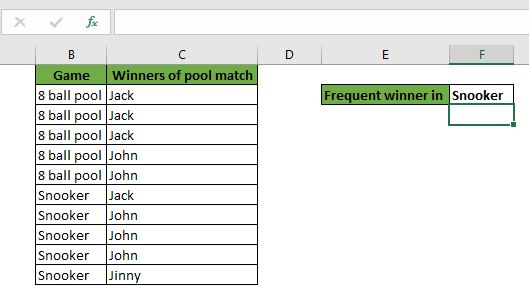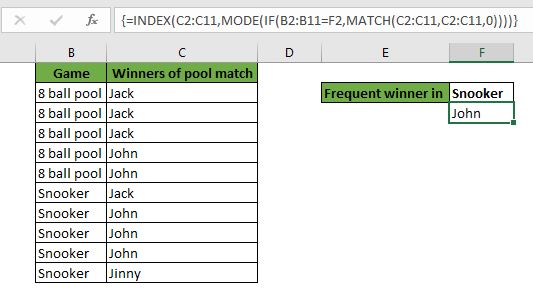# Lookup Frequently Appearing Text with Criteria in Excel

In the previous article, we learned how lookup frequently appearing text in range. In this article, we will learn how to lookup frequently appearing text with criteria.

Generic Formula

 =INDEX(range,MODE(IF(criteria range=criteria,MATCH(range,range,0))))

Range: This is the range from which you want to lookup frequently appearing text.

Criteria range: It is the range from which you want to put criteria on.

Criteria: the criteria that you want to put.

Let's see an example to see how we can retrieve frequently appearing text with the condition in Excel.

Example: Get the name of the player from each year that wins most of the matches.Here, I have a record of 8 ball pool and snooker matches. We want to look up the name of the frequent winner in snooker.

Write this formula in F3 and copy it in the right of the cell.

 =INDEX(C2:C11,MODE((B2:B11=F2,MATCH(C2:C11,C2:C11,0))))

As you can see, the most frequent names are retrieved in the cells.How does it work?

Here, we have used a combination of INDEX, MODE , IF and MATCH function.

As usual, the formula starts working from the first IF function.

The if function checks the criteria in the given range. Here it range is B2:B11 and criteria is "snooker". This returns array {FALSE;FALSE;FALSE;FALSE;FALSE;TRUE;TRUE;TRUE;TRUE;TRUE}. For each true, the match function runs.

Next, to MATCH function we have supplied the same C2:C11 range as the lookup value and lookup array with an exact match. This allows the MATCH function to return an array. The array we get indexes of the first appearance of each value. This means if Jack has appeared on index 1 then on every appearance of jack array will have 1. In this case, we get an array {FALSE;FALSE;FALSE;FALSE;FALSE;1;4;4;4;10}.

Next, this array is encapsulated in the MODE function. The MODE function returns the most frequently appearing number. In our case, the MODE function returns 4. This will be the row number for the INDEX function.

Next, we have the INDEX function that has an array as C2:B1a and the row number is 4 to retrieve the value from. Hence, we got John for the year 2016.

Note: This formula works in a one-dimensional range only. I mean, the range you select must be rows only or columns only. If you provide a two-dimensional array/range, it will return #N/A.

So yeah guys, this is how you get the most frequently appearing text in range. This can be used in excel 2007, 2010, 2013, 2016, and 365. If you have any doubts regarding this article or any other excel topic, ask me in the comments section below.Lookup most frequently appearing text with criteria in Excel

Related Articles:

Lookup Frequently Appearing Text | Learn how to lookup frequently appearing text in excel.

Retrieving the First Value in a List that is Greater / Smaller than a Specified Value | Learn how to lookup first value that is greater than a specified value. The generic formula to lookup is...

How to retrieve the entire row of a matched value| It is easy to look up and retrieve the entire row from a table. Let's see how can we do this lookup.

How to Retrieve Latest Price in Excel | To look up the latest price from a range you must have your data sorted. Once sorted use this formula...

Popular Articles:

50 Excel Shortcuts to Increase Your Productivity | Get faster at your task. These 50 shortcuts will make you work even faster on Excel.

The VLOOKUP Function in Excel | This is one of the most used and popular functions of excel that is used to lookup value from different ranges and sheets.

COUNTIF in Excel 2016 | Count values with conditions using this amazing function. You don't need filter your data to count specific value. Countif function is essential to prepare your dashboard.

How to Use SUMIF Function in Excel | This is another dashboard essential function. This helps you sum up values on specific conditions.

Terms and Conditions of use

The applications/code on this site are distributed as is and without warranties or liability. In no event shall the owner of the copyrights, or the authors of the applications/code be liable for any loss of profit, any problems or any damage resulting from the use or evaluation of the applications/code.